# 1 Affine transformations

On the set of all points of the space ${}_{\infty }{\mathbf{E}}^{n}\left(R\right)$ with defined homogeneous coordinate system, there is defined a group of affine transformations called affinities - affine mappings, or similarities. Affine transformations of the extended Euclidean space keep invariant one plane of the space, the ideal plane consisting of all ideal points in the space.

The ideal plane is not point invariant, it means, ideal points are mapped under affine transformations to ideal point only. Invariant property of all affine transformations (in addition to incidence) is therefore the Euclidean "parallelism", or common direction of lines, pointing to the same ideal point at infinity. Centre of a geometric figure is mapped to the centre of its image under the affine transformation. Size of line segments is no more invariant, therefore the size of geometric figures changes under these transformations. Image of a geometric figure (original) under an affine transformation is geometric figure similar to the original, keeping parallelism all parallel lines parallel also in the image.

Affine transformations of the space are: identity, scaling, shearing, axial affinity.

Affine transformations are analytically represented by regular square matrices of rank 4, while determinant of the matrix is nonzero, which means $|{\mathrm{T}}_{\mathrm{A}}|\ne 0$ and the matrix is in form

 ${\mathrm{T}}_{\mathrm{A}}=\left(\begin{array}{cccc}a& b& c& 0\\ d& e& f& 0\\ g& k& l& 0\\ m& n& p& s\end{array}\right)$

where entries of the matrix are coefficients of affine transformation with a clear geometric interpretation:

1. a, e, l are nonzero scales on coordinate axes x, y and z respectively
2. s is the scaling factor of the homothety through the origin O of the coordinate system
3. m, n, l are coordinates of translation vector
4. b, c, d, f, g, k are coefficients of a general affine transformation

## 1.1 Affine transformations in the plane

In the two-dimensional extended Euclidean space ${}_{\infty }{\mathbf{E}}^{2}\left(R\right)$ with the defined homogeneous coordinate system, a group of affine transformations can be determined. Any point $A=$$\left(x,y,{a}_{0}\right)$ for ${a}_{0}=0,\phantom{\rule{mediummathspace}{0.2em}}1$ in the plane can be attached its image $A\text{'}=\left(x\text{'},y\text{'},{a}_{0}\right)$, while the relation of coordinates can be expressed in the following way

 $A\text{'}=A.{\mathrm{T}}_{\mathrm{A}}$

where ${\mathrm{T}}_{\mathrm{A}}\phantom{\rule{mediummathspace}{0.2em}}$is the regular square matrix of rank 3.

We recognize the following affine transformations of plane: identity, homothety, scaling, shearing, axial affinity.

### 1.1 Homothety

Homothety is a transformation scaling all coordinates of points by an overall nonzero scale factor, it means in the same ratio, with respect to a given centre of homothety.

Let the homothety be given by the centre in the origin O and scaling coefficient $r\ne 0$, then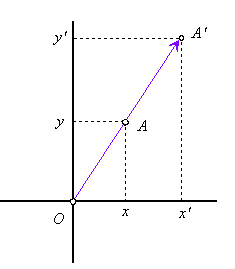Figure 1: Scaling through origin

Equations and matrix of homothety

 $x\text{'}=r\phantom{\rule{mediummathspace}{0.2em}}x$
 $y\text{'}=r\phantom{\rule{mediummathspace}{0.2em}}y$
 ${\mathrm{T}}_{\mathrm{A}}=\left(\begin{array}{ccc}r& 0& 0\\ 0& r& 0\\ 0& 0& 1\end{array}\right)\approx \left(\begin{array}{ccc}1& 0& 0\\ 0& 1& 0\\ 0& 0& s\end{array}\right),\phantom{\rule{mediummathspace}{0.2em}}\mathrm{while}\phantom{\rule{mediummathspace}{0.2em}}s=\frac{1}{r}\ne 0$

### 1.2 Scaling on coordinate axes

Scaling can be defined also on separate coordinate axes by a local scale factors, which are different nonzero coefficients.

Let the scaling be given by the scale factors $a,b\ne 0$, related to coordinate axes x and y respectively, then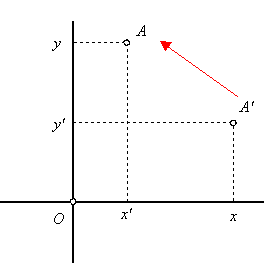Figure 2: Scaling on coordinate axes

Equations and matrix of scaling on coordinate axes

 $x\text{'}=a\phantom{\rule{mediummathspace}{0.2em}}x$
 $y\text{'}=b\phantom{\rule{mediummathspace}{0.2em}}y$
 ${\mathrm{T}}_{\mathrm{A}}=\left(\begin{array}{ccc}a& 0& 0\\ 0& b& 0\\ 0& 0& 1\end{array}\right)$

### 1.3 Shearing

Transformation of the plane under which points are moved in direction of one from the coordinate axes by vectors determined through nonzero coefficients is called shearing.

Let the shearing in direction of coordinate axis x be determined by coefficient c, then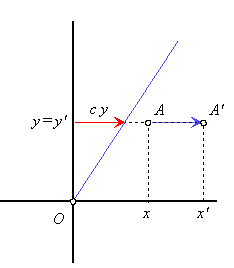Figure 3: Shearing in direction of axis x

Equations and matrix of shearing in direction of axis x

 $x\text{'}=x+c\phantom{\rule{mediummathspace}{0.2em}}y$
 $y\text{'}=y$
 ${\mathrm{T}}_{\mathrm{A}}=\left(\begin{array}{ccc}1& 0& 0\\ c& 1& 0\\ 0& 0& 1\end{array}\right)$

Let the shearing in direction of coordinate axis y be determined by coefficient b, then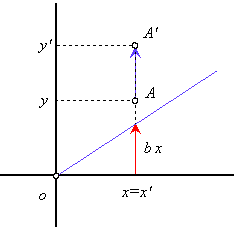Figure 4: Shearing in direction of axis y

Equations and matrix of shearing in direction of axis y

 $x\text{'}=x$
 $y\text{'}=b\phantom{\rule{mediummathspace}{0.2em}}x+y$
 ${\mathrm{T}}_{\mathrm{A}}=\left(\begin{array}{ccc}1& b& 0\\ 0& 1& 0\\ 0& 0& 1\end{array}\right)$

### 1.4 Axial affinity

Axial affinity is transformation of the plane, which keeps point invariant one line in the plane, and one pencil of lines not as point invariant.

Let the axial affinity be determined by the invariant line - axis of affinity in the coordinate axis x, and a pair of corresponding points $A=\left(a,b,1\right)↦A\text{'}=\left(c,d,1\right)$ representing the direction $\mathbf{s}=\stackrel{\to }{A\phantom{\rule{mediummathspace}{0.2em}}A\text{'}}=\left(c-a,d-b,0\right)$ of the invariant pencil of lines, then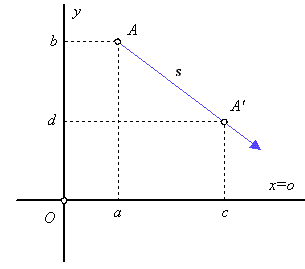Figure 5: Axial affinity

Equations and matrix of axial affinity

 $x\text{'}=\phantom{\rule{mediummathspace}{0.2em}}x+\frac{c-a}{b}\phantom{\rule{mediummathspace}{0.2em}}y$
 $y\text{'}=\frac{d}{b}\phantom{\rule{mediummathspace}{0.2em}}y$
 ${\mathrm{T}}_{\mathrm{A}}=\left(\begin{array}{ccc}b& 0& 0\\ c-a& d& 0\\ 0& 0& b\end{array}\right)\approx \left(\begin{array}{ccc}1& 0& 0\\ \frac{c-a}{b}& \frac{d}{b}& 0\\ 0& 0& 1\end{array}\right)$

## 2 Affine transformations in the space

In the three-dimensional extended Euclidean space ${}_{\infty }{\mathbf{E}}^{3}\left(R\right)$ with the defined homogeneous coordinate system, a group of affine transformations can be determined. Any point $A=$$\left(x,\phantom{\rule{2mm}{2mm}}y,\phantom{\rule{2mm}{2mm}}z,{a}_{0}\right)$ for ${a}_{0}=0,\phantom{\rule{mediummathspace}{0.2em}}1$ in the space can be attached its image $A\text{'}=\left(x\text{'},\phantom{\rule{2mm}{2mm}}y\text{'},{\phantom{\rule{2mm}{2mm}}z\text{'},\phantom{\rule{2mm}{2mm}}a}_{0}\right)$, while the relation of coordinates can be expressed in the following way

 $A\text{'}=A.{\mathrm{T}}_{\mathrm{A}}$

where ${\mathrm{T}}_{\mathrm{A}}\phantom{\rule{mediummathspace}{0.2em}}$is the regular square matrix of rank 4.

We recognize the following affine transformations of plane: identity, homothety, scaling, shearing, axial affinity, perspective affinity.

### 2.1 Homothety

Homothety is a transformation scaling all coordinates of points by an overall nonzero scale factor, it means in the same ratio, with respect to a given centre of homothety.

Let the homothety be given by the centre in the origin O and scaling coefficient $h\ne 0$, then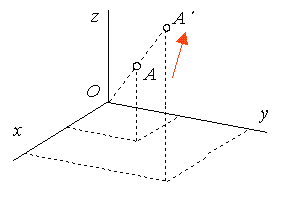Figure 6: Scaling through origin

Equations and matrix of homothety through origin

 $x\text{'}=h\phantom{\rule{mediummathspace}{0.2em}}x$
 $y\text{'}=h\phantom{\rule{mediummathspace}{0.2em}}y$
 $z\text{'}=h\phantom{\rule{mediummathspace}{0.2em}}z$
 ${\mathrm{T}}_{\mathrm{A}}=\left(\begin{array}{cccc}h& 0& 0& 0\\ 0& h& 0& 0\\ 0& 0& h& 0\\ 0& 0& 0& 1\end{array}\right)\approx \left(\begin{array}{cccc}1& 0& 0& 0\\ 0& 1& 0& 0\\ 0& 0& 1& 0\\ 0& 0& 0& s\end{array}\right),\phantom{\rule{mediummathspace}{0.2em}}s=\frac{1}{h}\ne 0$

### 2.2 Scaling

Scaling can be defined also on separate coordinate axes by a local scale factors, which are different nonzero coefficients.

Let the scaling be given by the scale factors $a,b,\phantom{\rule{mediummathspace}{0.2em}}c\ne 0$, related to coordinate axes x, y and z respectively, then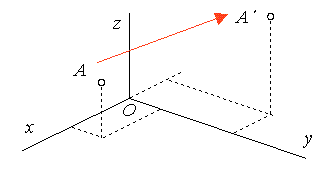Figure 7: Scaling on coordinate axes

Equations and matrix of scaling on coordinate axes

 $x\text{'}=a\phantom{\rule{mediummathspace}{0.2em}}x$
 $y\text{'}=b\phantom{\rule{mediummathspace}{0.2em}}y$
 $z\text{'}=c\phantom{\rule{mediummathspace}{0.2em}}z$
 ${T}_{A}=\left(\begin{array}{cccc}a& 0& 0& 0\\ 0& b& 0& 0\\ 0& 0& c& 0\\ 0& 0& 0& 1\end{array}\right)$

### 2.3 Shearing

Transformation of the space under which points are moved in direction of one from the coordinate axes by vectors determined through nonzero coefficients is called shearing.

Let the shearing in direction of coordinate axis z be determined by coefficients c, b, then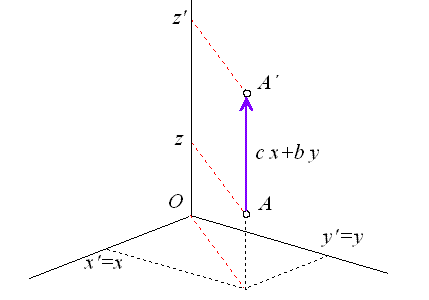Figure 8: Shearing in direction of axis z

Equations and matrix of shearing in direction of axis z

 $x\text{'}=x$
 $y\text{'}=y$
 $z\text{'}=z+c\phantom{\rule{mediummathspace}{0.2em}}x+b\phantom{\rule{mediummathspace}{0.2em}}y$
 ${T}_{A}=\left(\begin{array}{cccc}1& 0& c& 0\\ 0& 1& b& 0\\ 0& 0& 1& 0\\ 0& 0& 0& 1\end{array}\right)$

### 2.4 Perspective affinity

Perspective affinity is transformation of the space, which keeps point invariant one line in the space, and invariant one pencil of lines but not point invariant.

Let the perspective affinity be determined by the invariant line - axis of affinity in the coordinate axis x, and a pair of corresponding points $A=\left(a,b,0,1\right)↦A\text{'}=\left(c,d,e,1\right)$ representing the direction $\mathbf{s}=\stackrel{\to }{A\phantom{\rule{mediummathspace}{0.2em}}A\text{'}}=\left(c-a,d-b,e,0\right)$ of the invariant pencil of lines, then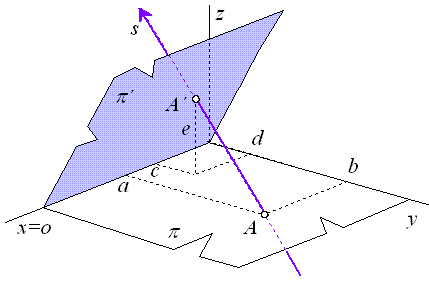Figure 9: Perspective affinity

Equations and matrix of perspective affinity

 $x\text{'}=x+\frac{c-a}{b}\phantom{\rule{2mm}{2mm}}y$
 $y\text{'}=\frac{d}{b}\phantom{\rule{2mm}{2mm}}y$
 $z\text{'}=\frac{e}{b}\phantom{\rule{2mm}{2mm}}y+z$
 ${\mathrm{T}}_{\mathrm{A}}=\left(\begin{array}{cccc}b& 0& 0& 0\\ c-a& d& e& 0\\ 0& 0& b& 0\\ 0& 0& 0& b\end{array}\right)=\left(\begin{array}{cccc}1& 0& 0& 0\\ \frac{c-a}{b}& \frac{d}{b}& \frac{e}{b}& 0\\ 0& 0& 1& 0\\ 0& 0& 0& 1\end{array}\right)$